# IV Projection A projection transformation moves from three

• Slides: 86IV Projection • A projection transformation moves from three dimensions to two dimensions • Projections occur based on the viewpoint and the viewing direction • Projections move objects onto a projection plane • Projections are classified based on the direction of projection, the projection plane normal, the view direction, and the viewpoint • Two primary classifications are parallel and perspective 1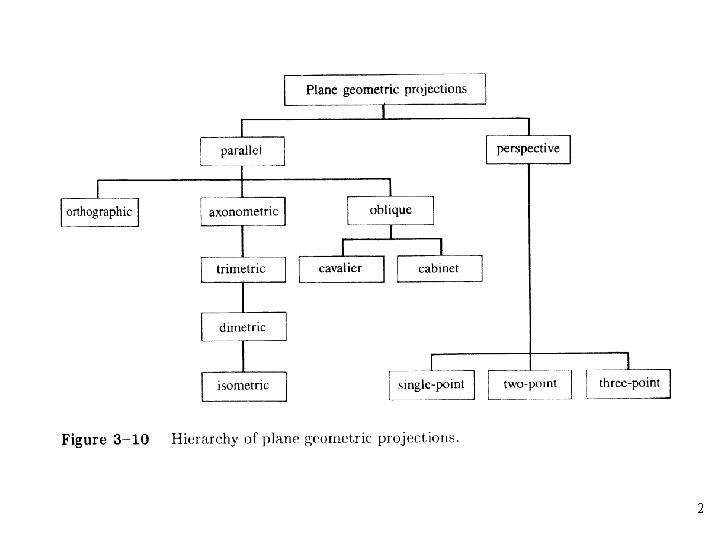23Parallel Projection • All projectors run parallel and in the viewing direction • Projecting onto the z = 0 plane along the z axis results in all z coordinates being set to zero 4Orthographic Projection • The simplest of the parallel projections, which is commonly used for engineering drawings • They accurately show the correct or true size and shape of a single plane face of an object • The matrix for projection onto the x=0 plane • The matrix for projection onto the y=0 plane 5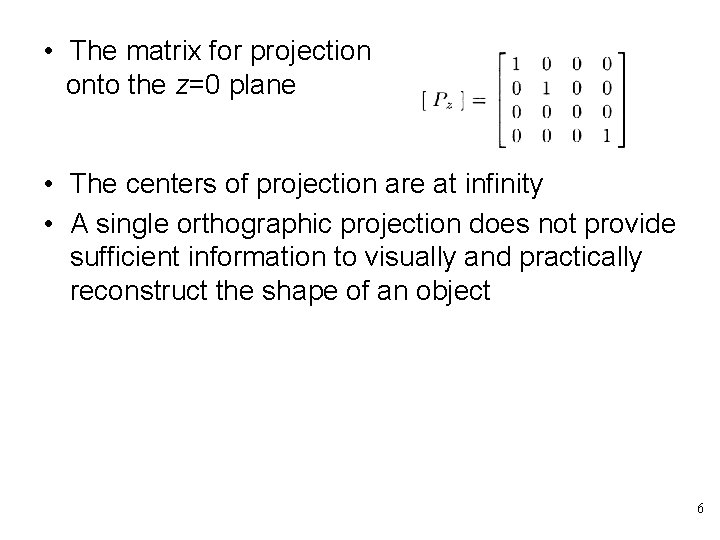• The matrix for projection onto the z=0 plane • The centers of projection are at infinity • A single orthographic projection does not provide sufficient information to visually and practically reconstruct the shape of an object 6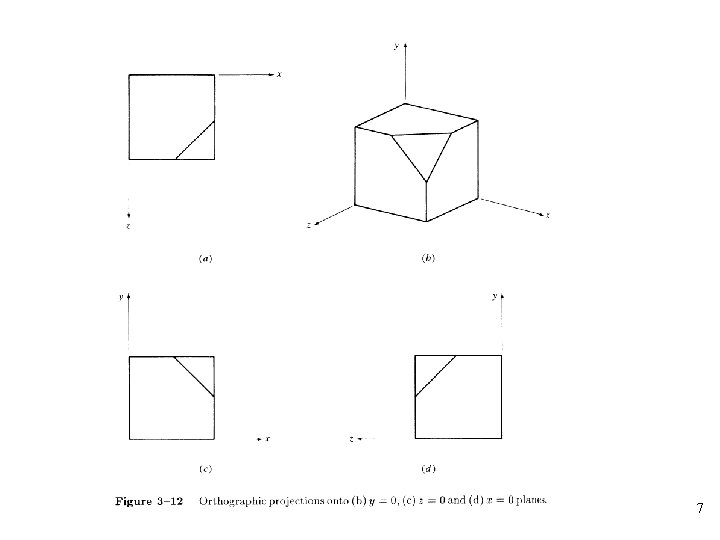7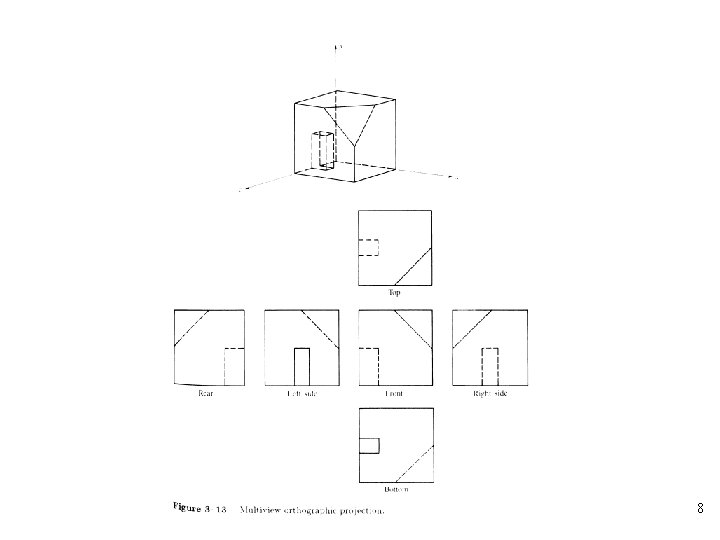89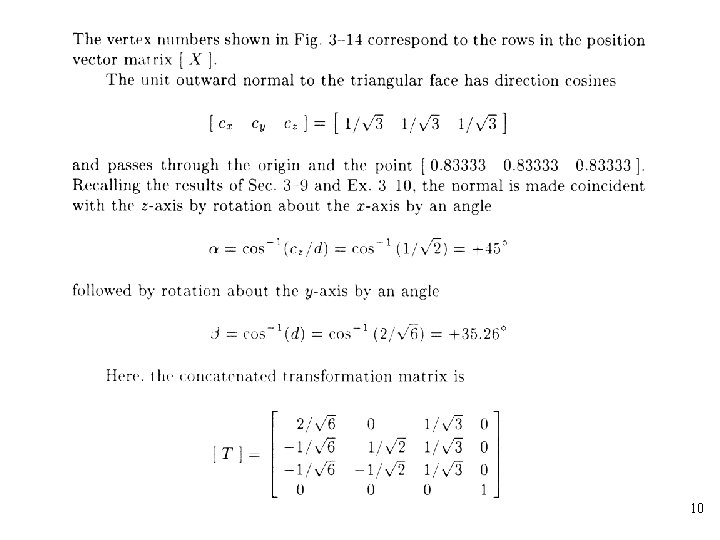101112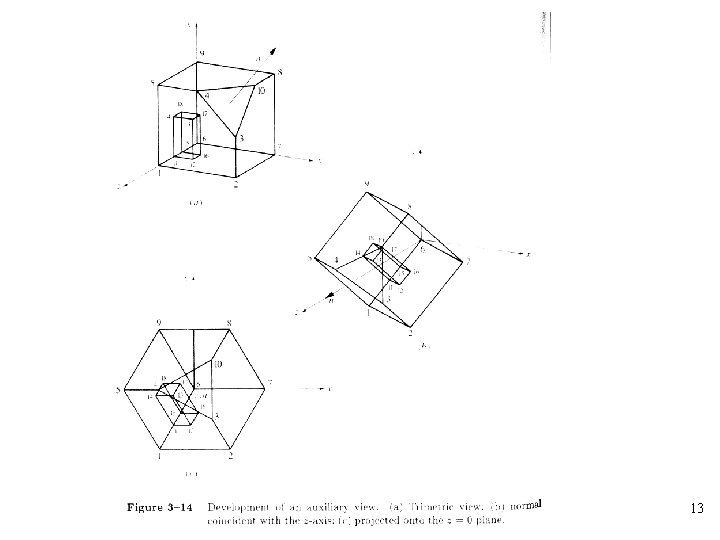13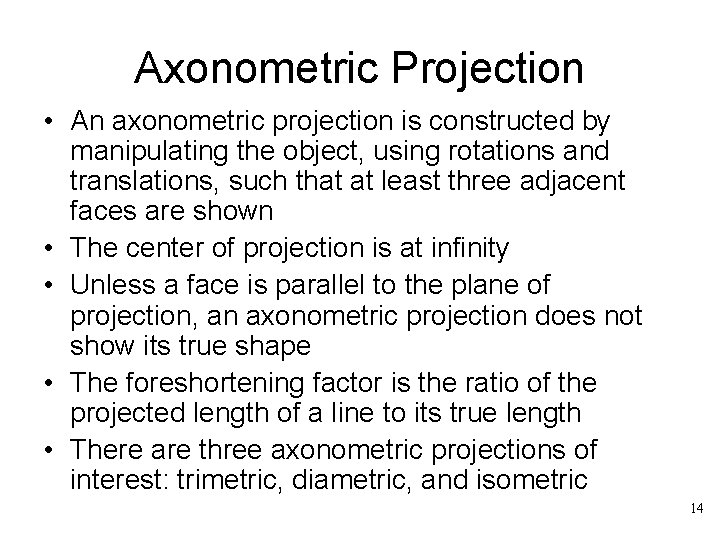Axonometric Projection • An axonometric projection is constructed by manipulating the object, using rotations and translations, such that at least three adjacent faces are shown • The center of projection is at infinity • Unless a face is parallel to the plane of projection, an axonometric projection does not show its true shape • The foreshortening factor is the ratio of the projected length of a line to its true length • There are three axonometric projections of interest: trimetric, diametric, and isometric 14• An isometric projection is a special case of a diametric projection, and a diametric projection is a special case of a trimetric projection • Foreshortening factors along the projected principal axes are: • A trimetric projection is formed by arbitrary rotations, in arbitrary order, about any or all of the coordinate axes, followed by parallel projection onto the z=0 plane 151617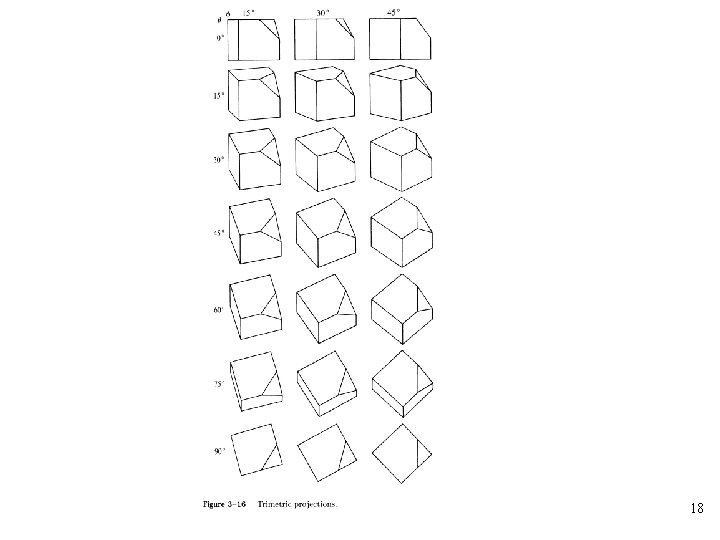18• A dimetric projection is a trimetric projection with two of the three foreshortening factors equal; the third is arbitrary • For example, a dimetric projection is constructed by a rotation about the y-axis through an angle followed by rotation about the x-axis through an angle and projection from a center of projection at infinity onto the z=0 plane: 19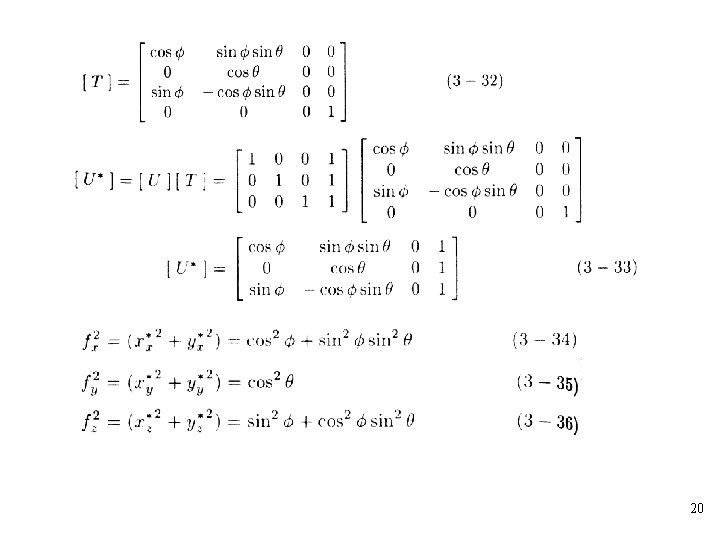20Let 2122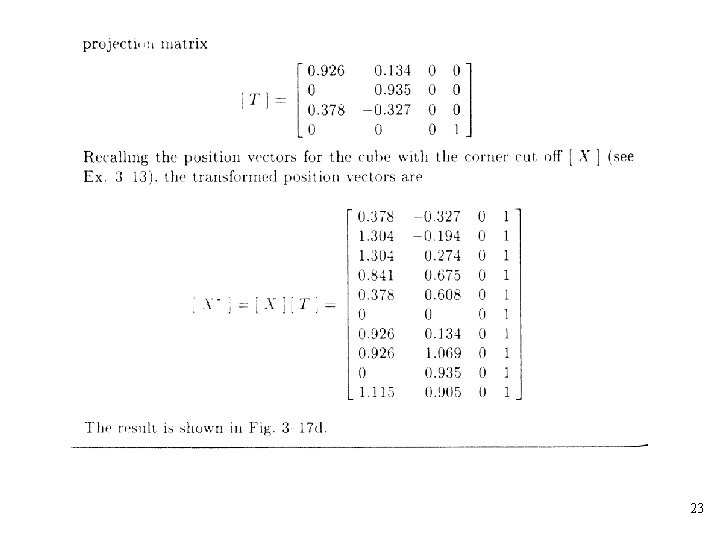23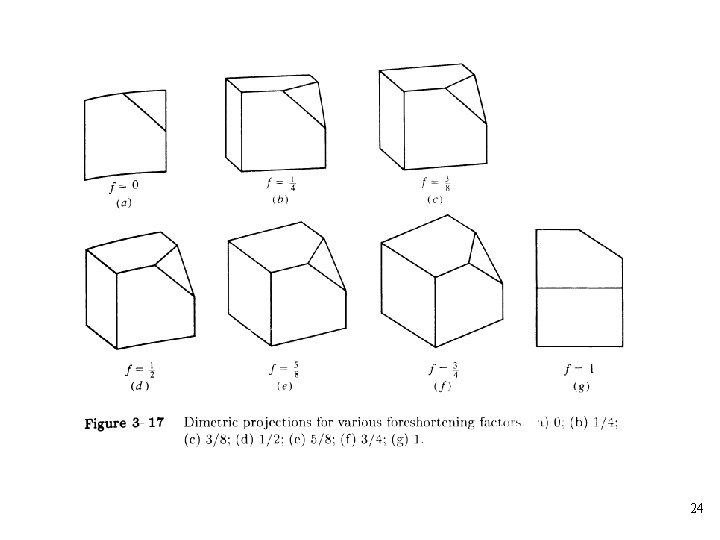2425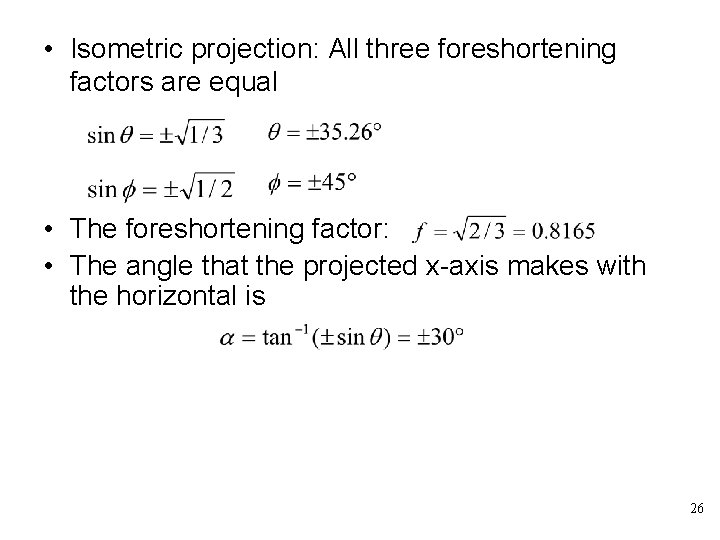• Isometric projection: All three foreshortening factors are equal • The foreshortening factor: • The angle that the projected x-axis makes with the horizontal is 26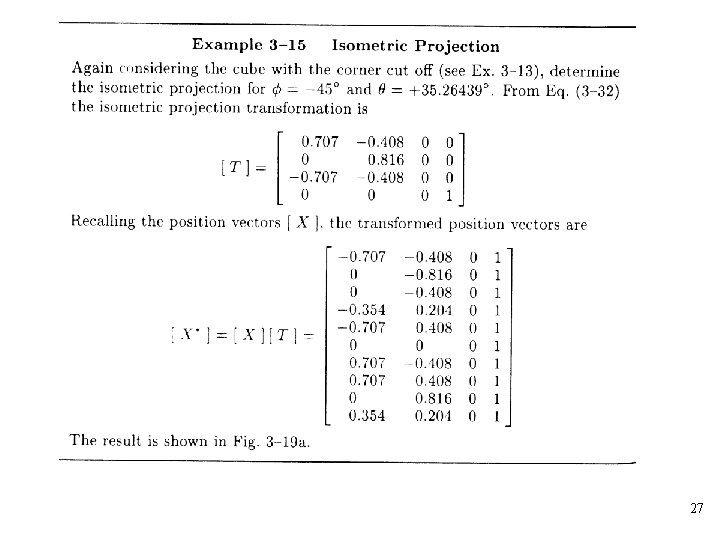27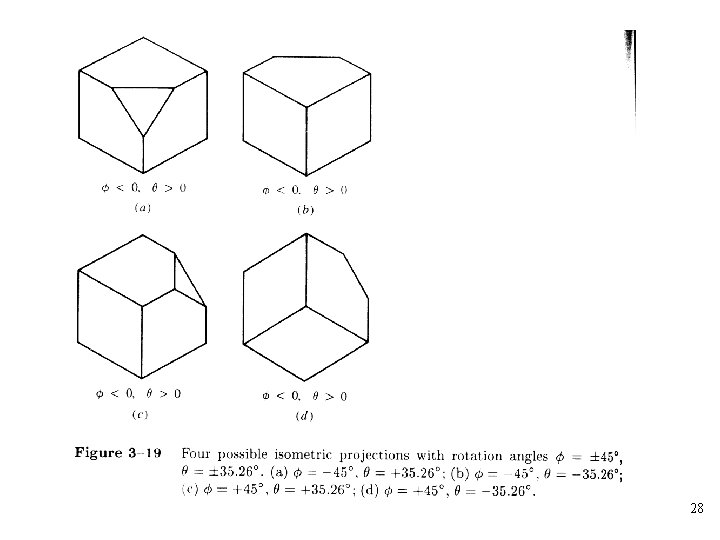28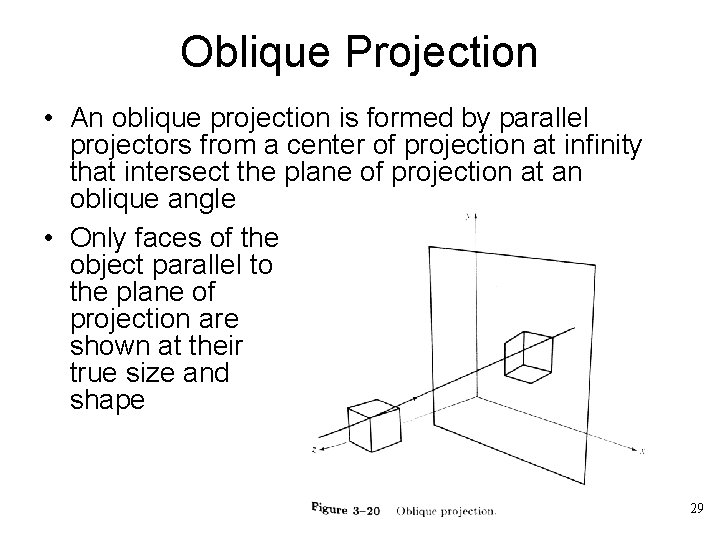Oblique Projection • An oblique projection is formed by parallel projectors from a center of projection at infinity that intersect the plane of projection at an oblique angle • Only faces of the object parallel to the plane of projection are shown at their true size and shape 29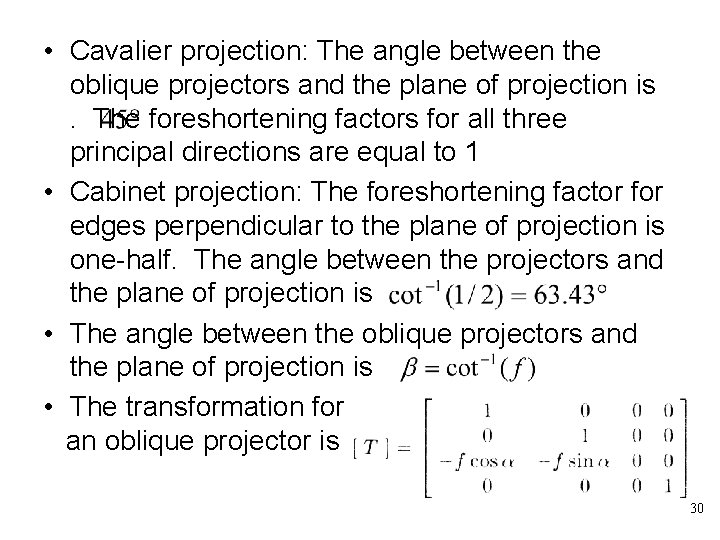• Cavalier projection: The angle between the oblique projectors and the plane of projection is. The foreshortening factors for all three principal directions are equal to 1 • Cabinet projection: The foreshortening factor for edges perpendicular to the plane of projection is one-half. The angle between the projectors and the plane of projection is • The angle between the oblique projectors and the plane of projection is • The transformation for an oblique projector is 30313233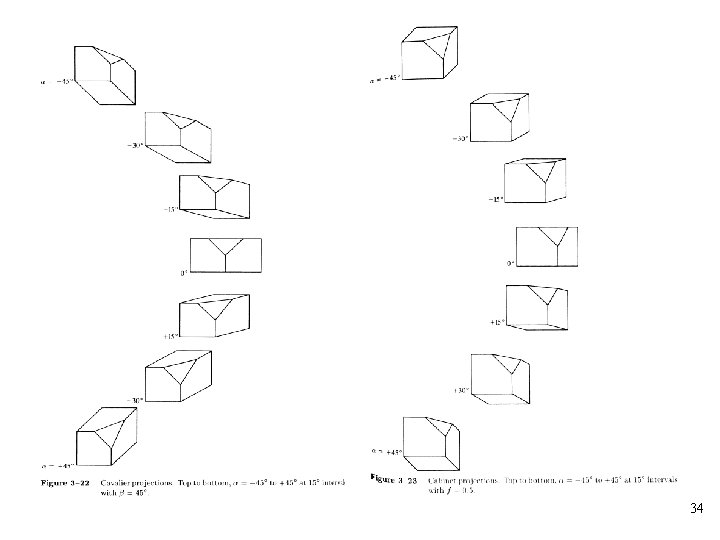34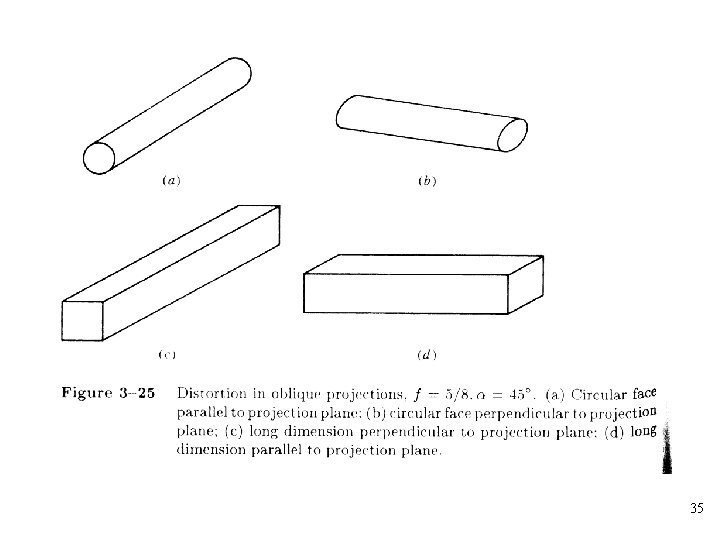35Perspective Projection • The viewpoint is the center of projection for a perspective projection • In perspective transformations parallel lines converge, object size is reduced with increasing distance from the center of projection • All of these effects aid the depth perception of the human visual system, but the shape of the object is not preserved 36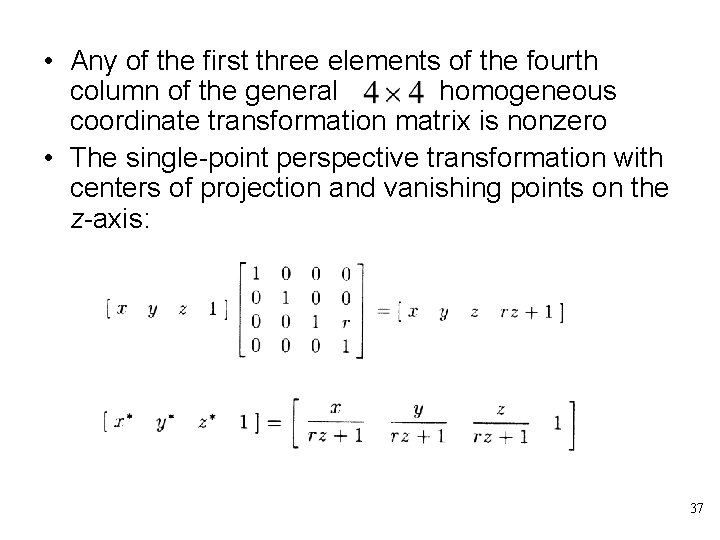• Any of the first three elements of the fourth column of the general homogeneous coordinate transformation matrix is nonzero • The single-point perspective transformation with centers of projection and vanishing points on the z-axis: 37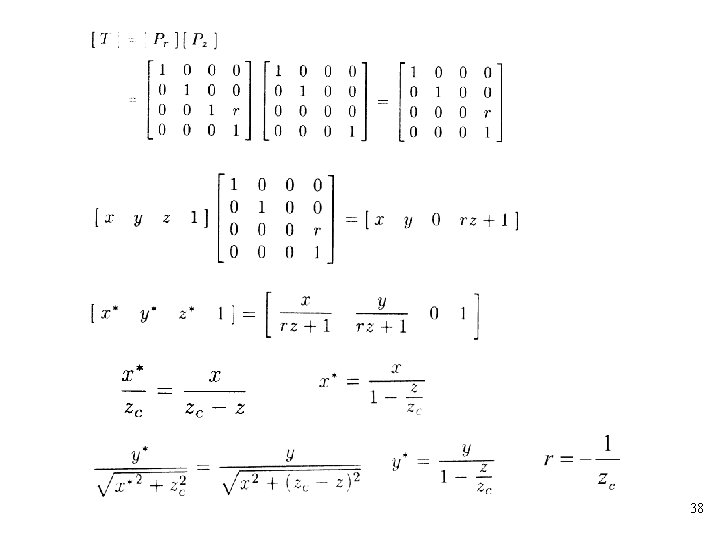38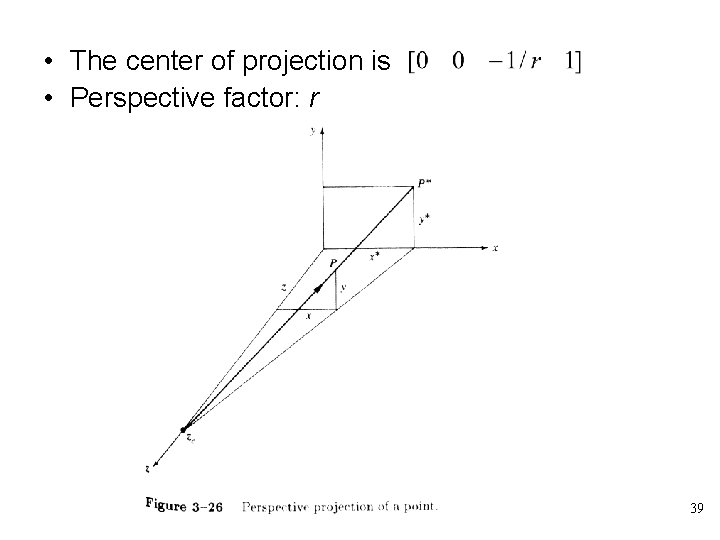• The center of projection is • Perspective factor: r 3940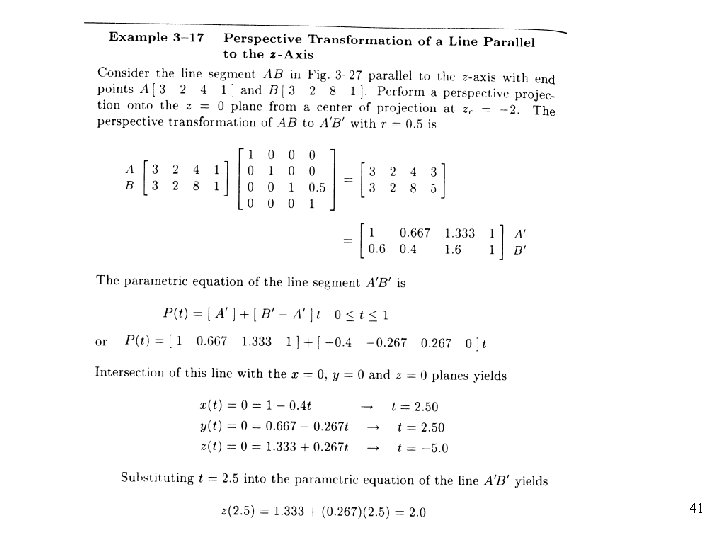41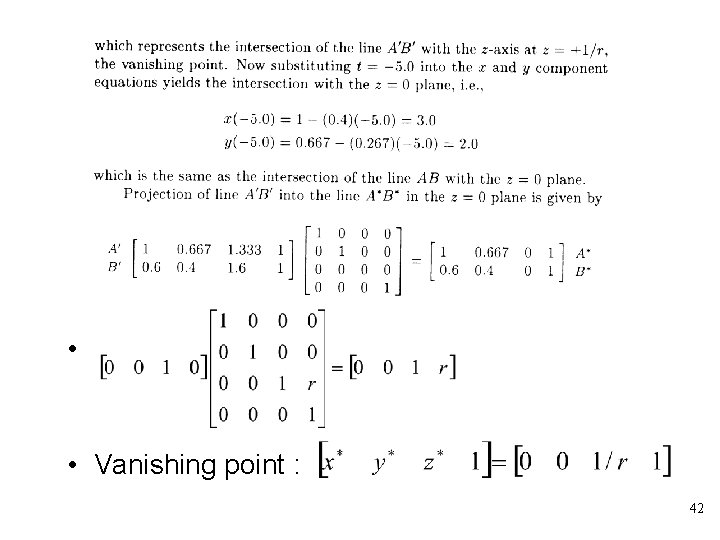• • Vanishing point : 42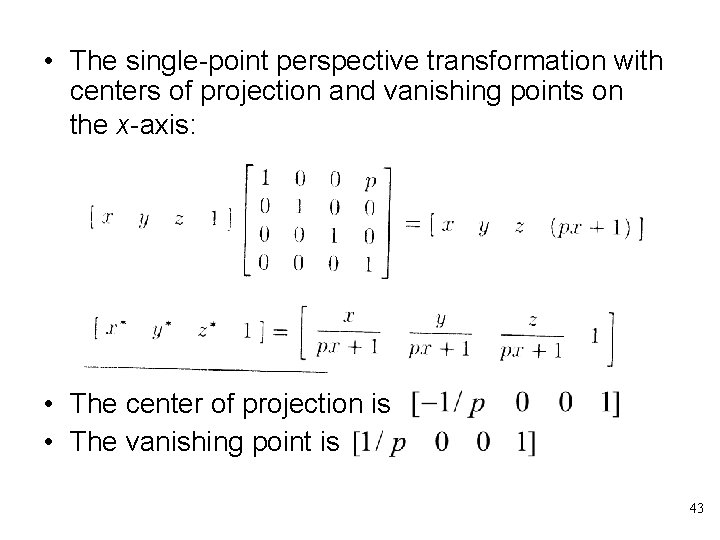• The single-point perspective transformation with centers of projection and vanishing points on the x-axis: • The center of projection is • The vanishing point is 43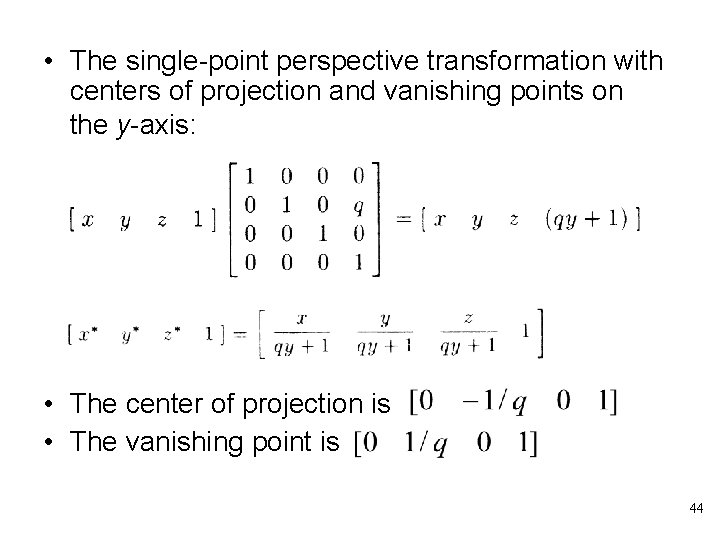• The single-point perspective transformation with centers of projection and vanishing points on the y-axis: • The center of projection is • The vanishing point is 44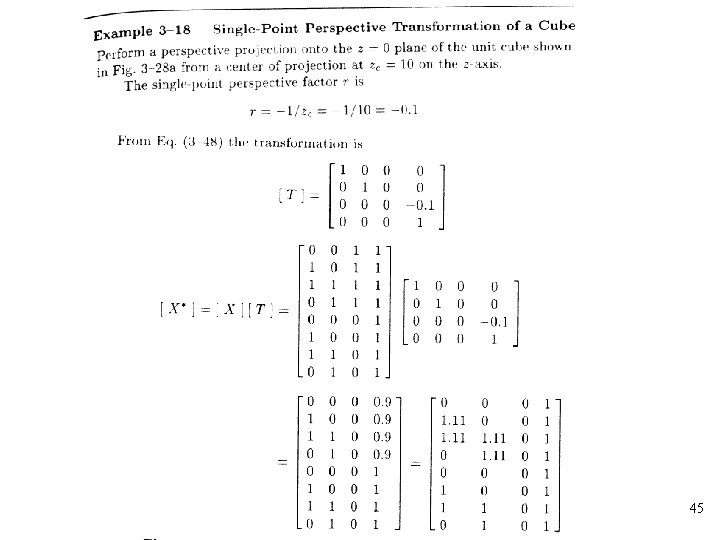45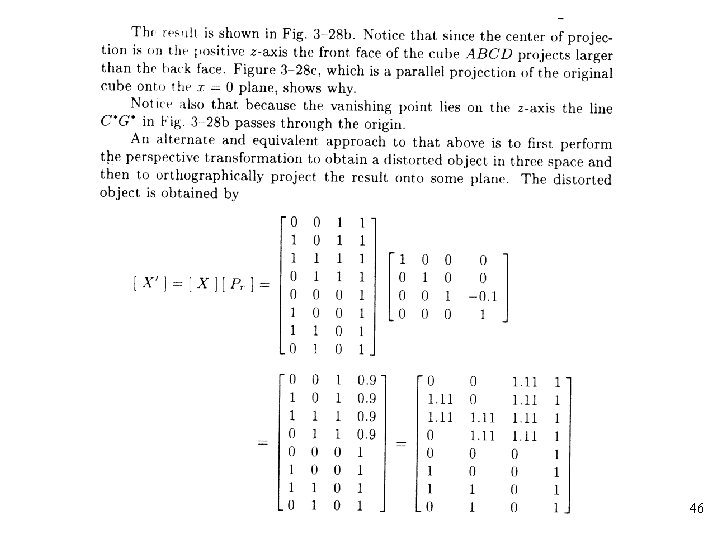46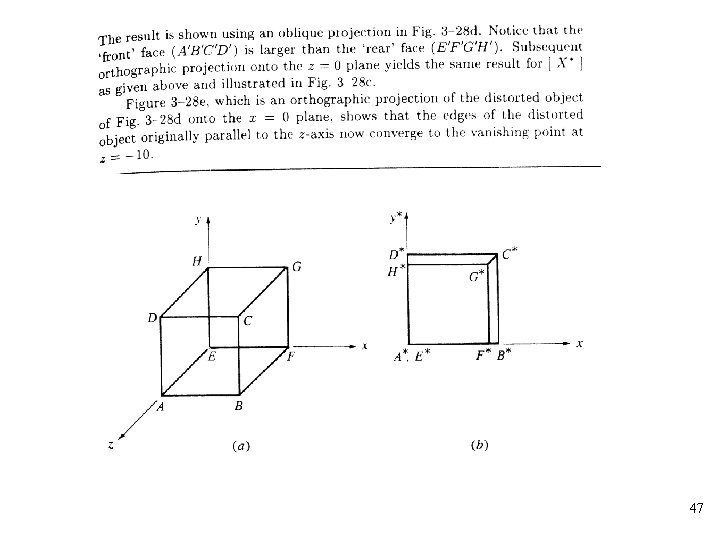4748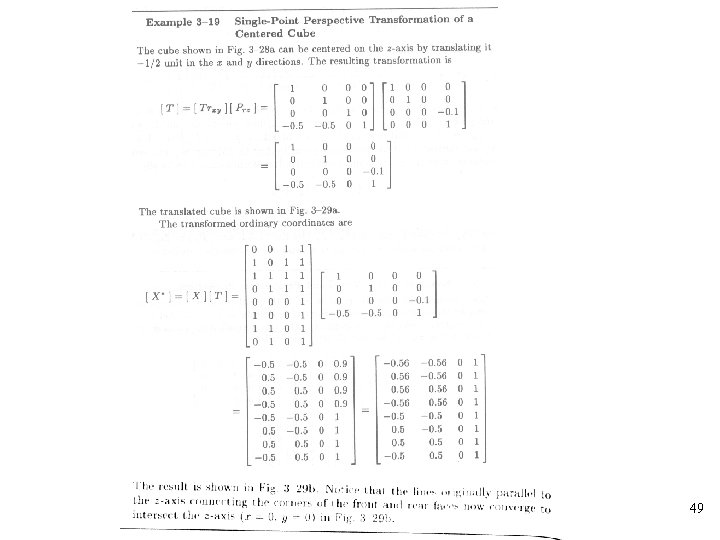49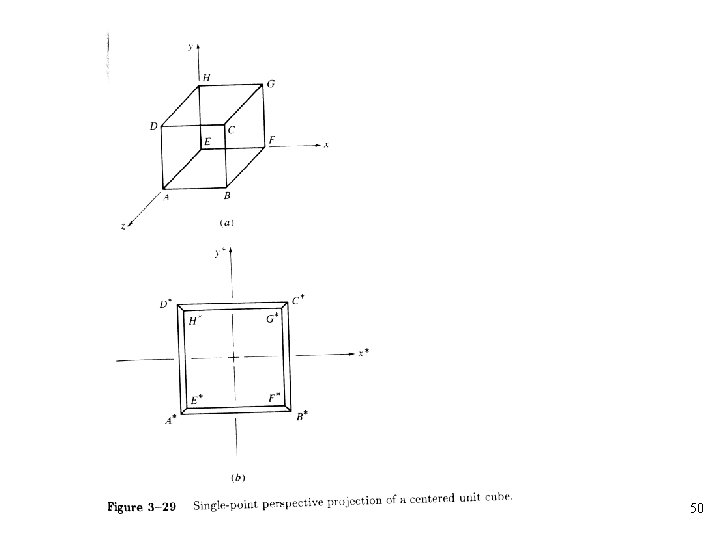50• Two-point perspective transformation: Two terms in the first three rows of the fourth column of the transformation matrix are nonzero. • Two centers of projection: • Two vanishing points: 515253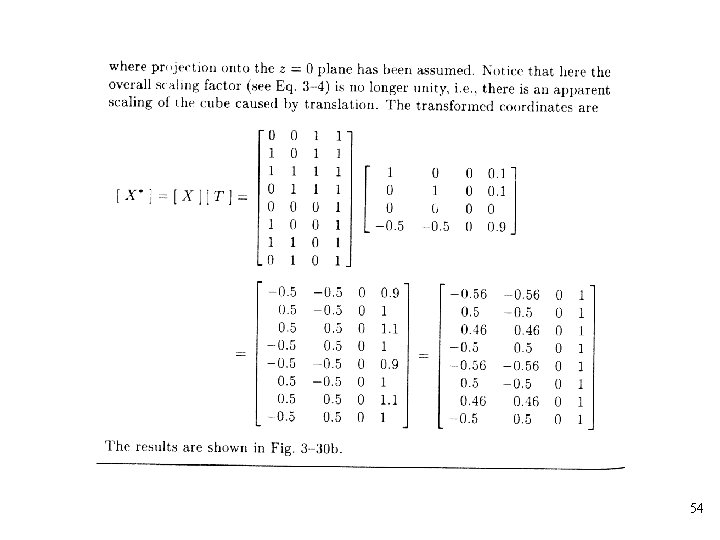5455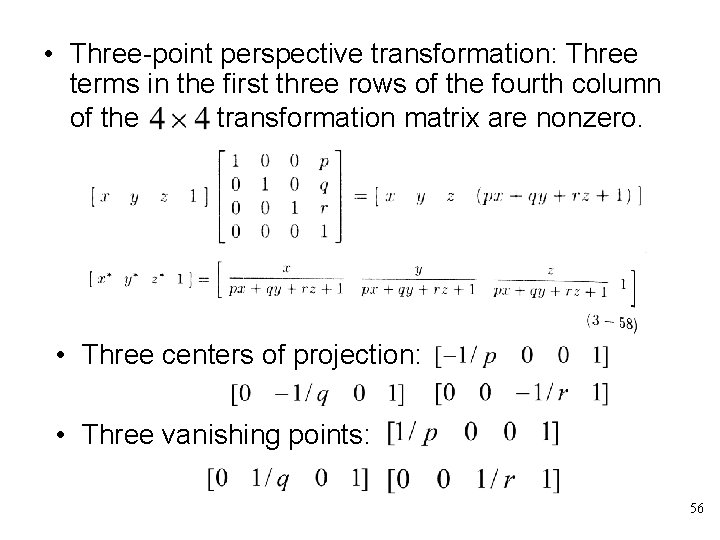• Three-point perspective transformation: Three terms in the first three rows of the fourth column of the transformation matrix are nonzero. • Three centers of projection: • Three vanishing points: 56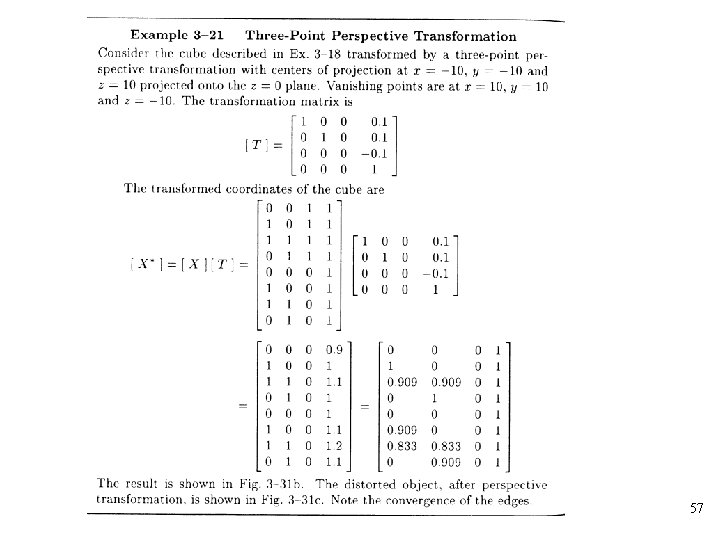5758• Consider simple translation of the object followed by a single-point perspective projection from a center of projection at onto the z=0 plane 59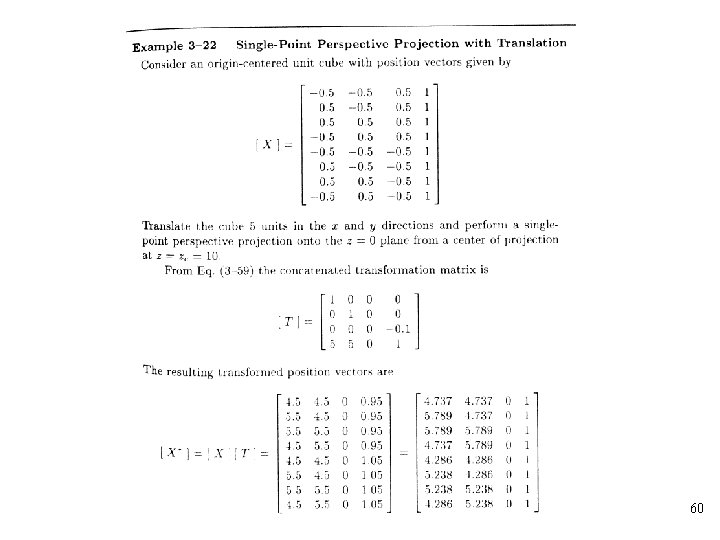60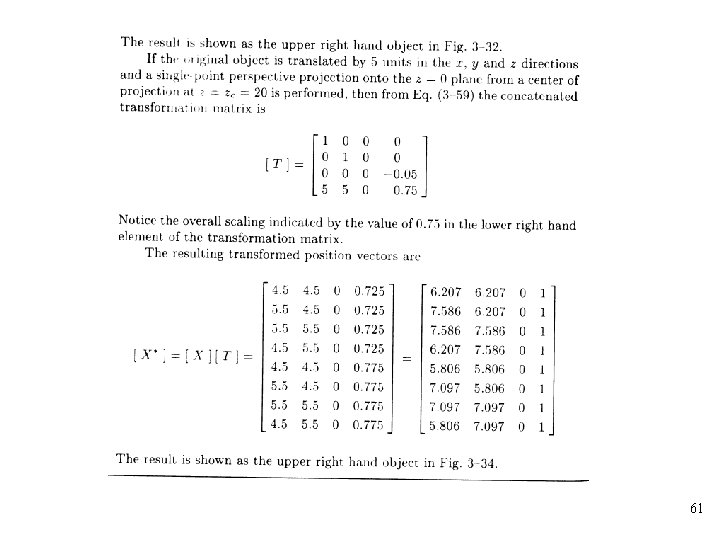616263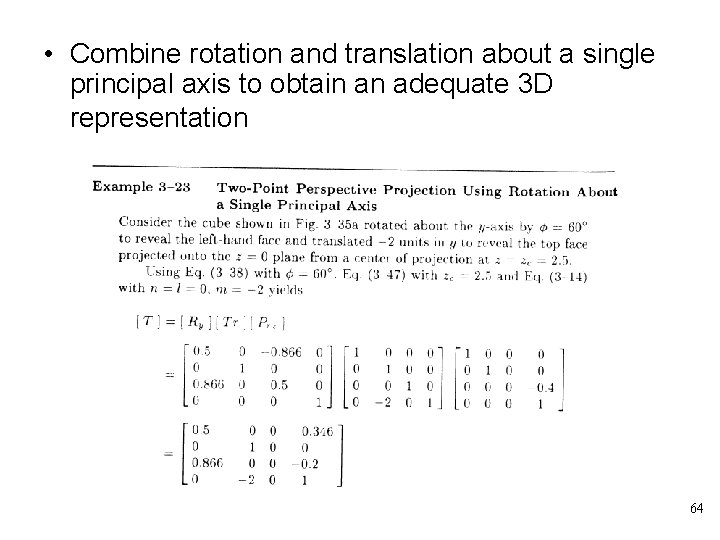• Combine rotation and translation about a single principal axis to obtain an adequate 3 D representation 64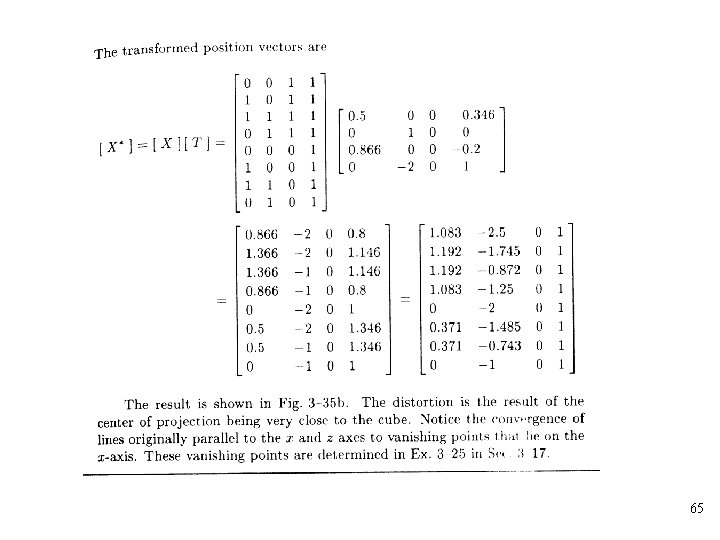65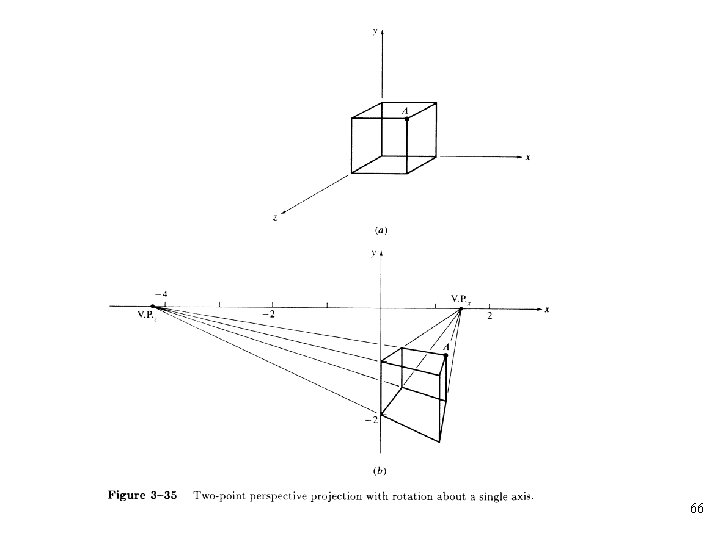66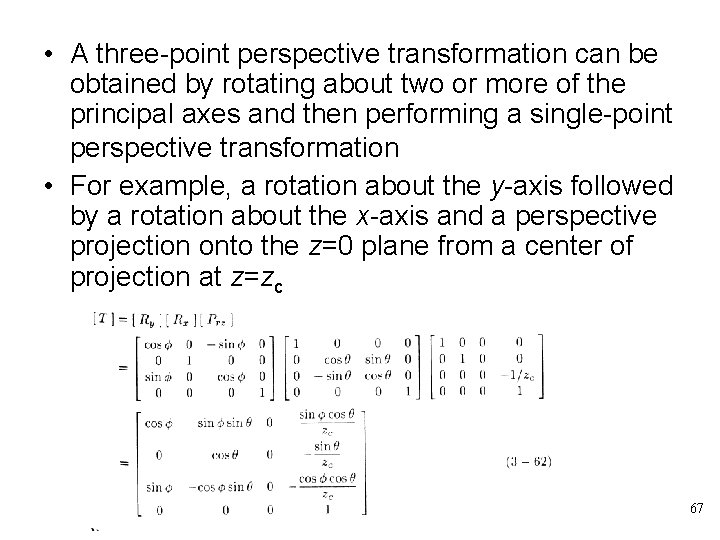• A three-point perspective transformation can be obtained by rotating about two or more of the principal axes and then performing a single-point perspective transformation • For example, a rotation about the y-axis followed by a rotation about the x-axis and a perspective projection onto the z=0 plane from a center of projection at z=zc 67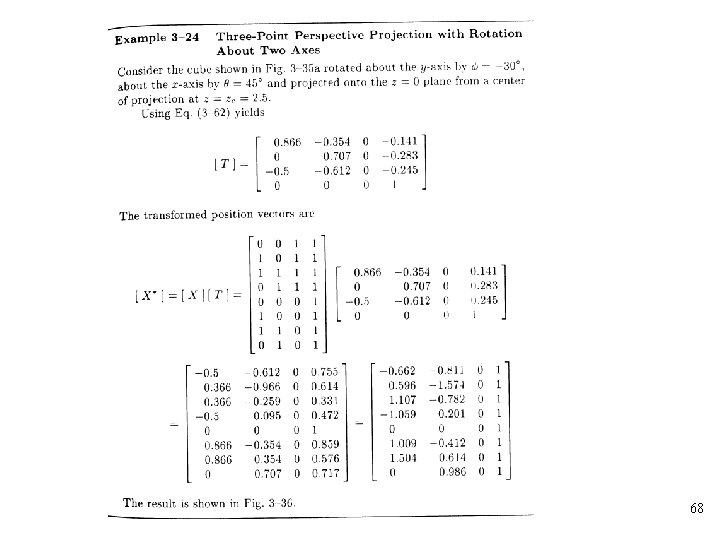68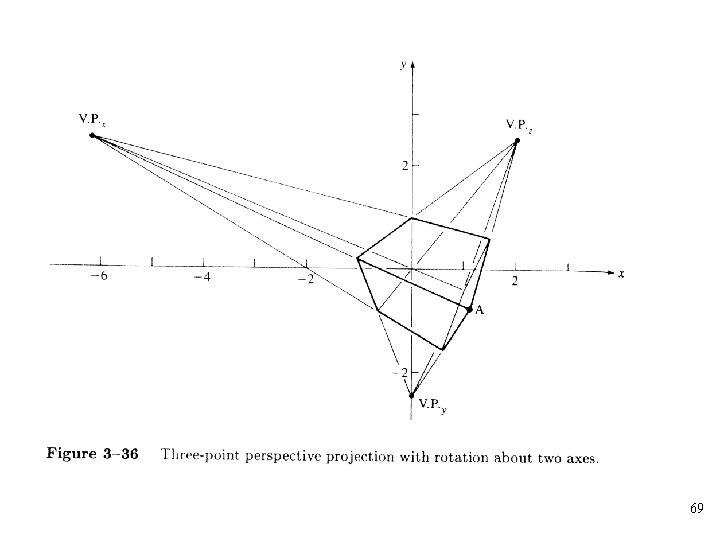69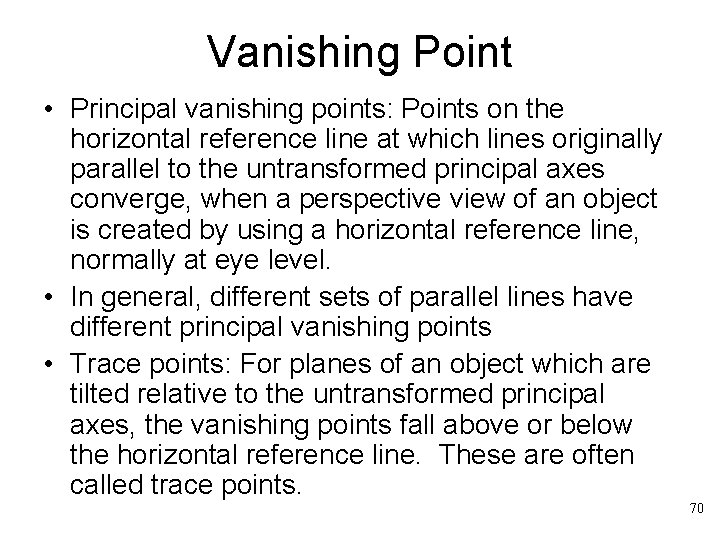Vanishing Point • Principal vanishing points: Points on the horizontal reference line at which lines originally parallel to the untransformed principal axes converge, when a perspective view of an object is created by using a horizontal reference line, normally at eye level. • In general, different sets of parallel lines have different principal vanishing points • Trace points: For planes of an object which are tilted relative to the untransformed principal axes, the vanishing points fall above or below the horizontal reference line. These are often called trace points. 70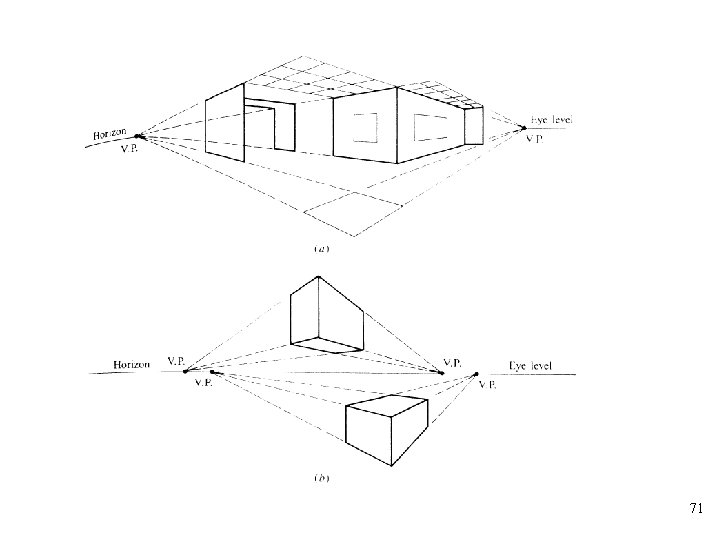71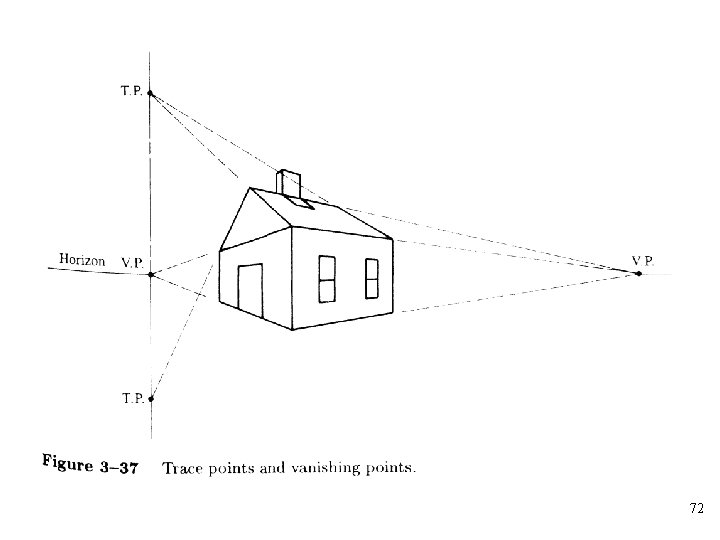72• The methods for determining the vanishing points: – The intersection point of a pair of transformed projected parallel lines 73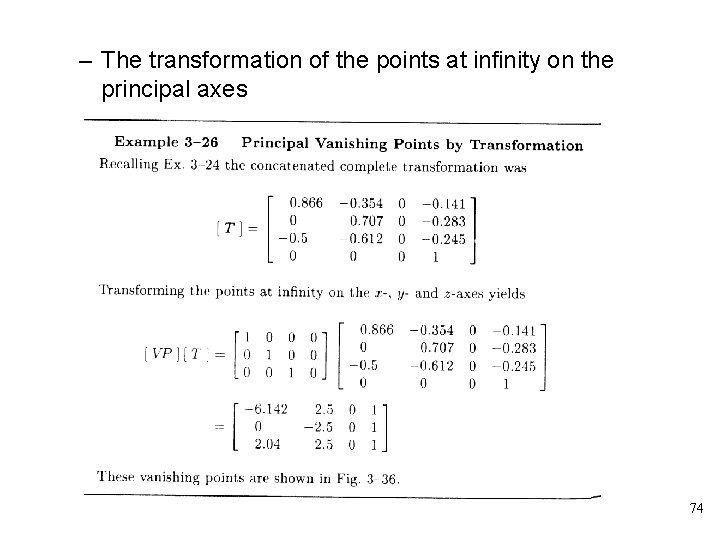– The transformation of the points at infinity on the principal axes 74• The transformation of the points at infinity for skew planes can be used to find trace point 7576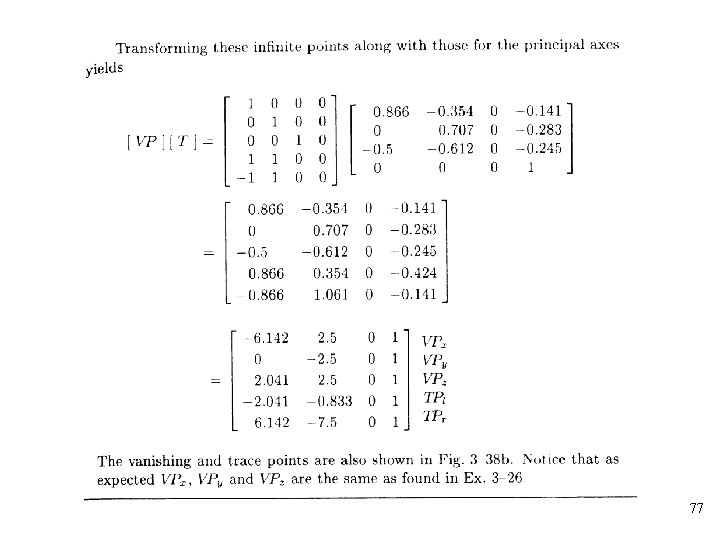77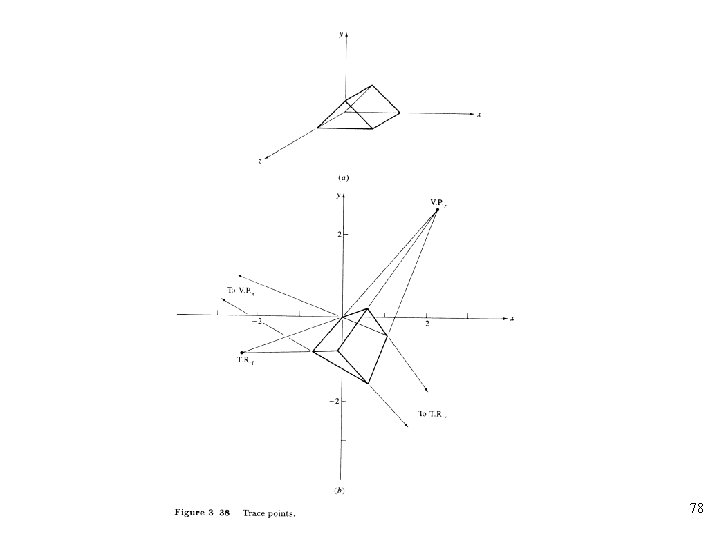78Reconstruction of 3 D Images • The general perspective transformation is: • The transformation projected onto the z=0 plane is 79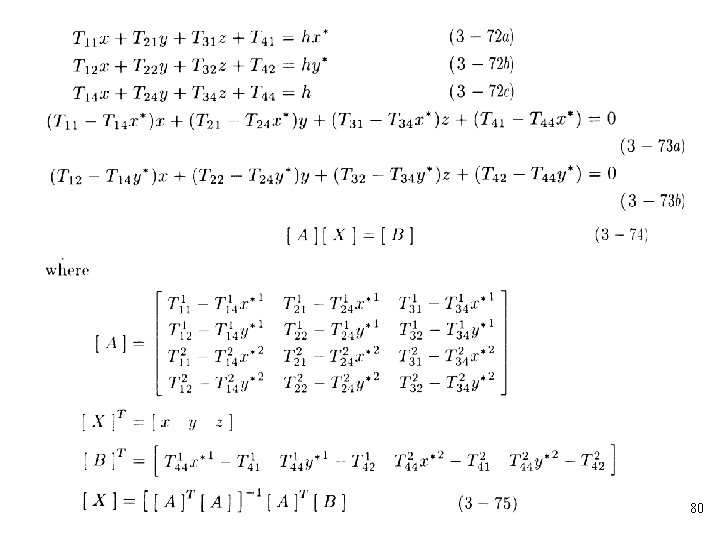80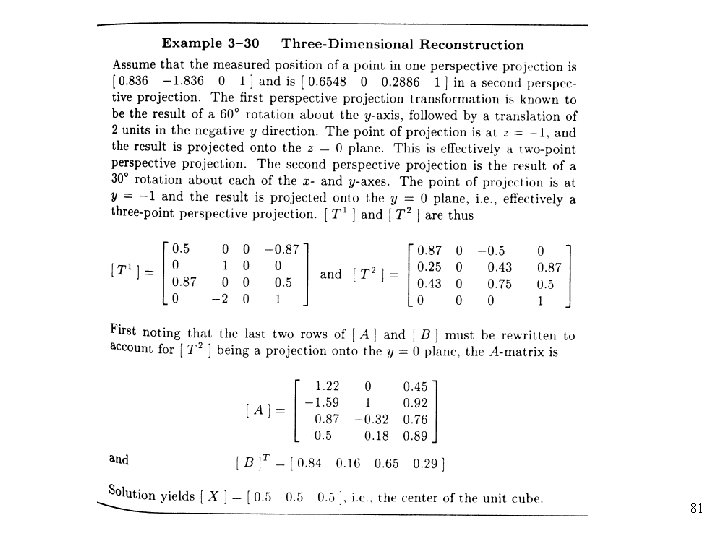81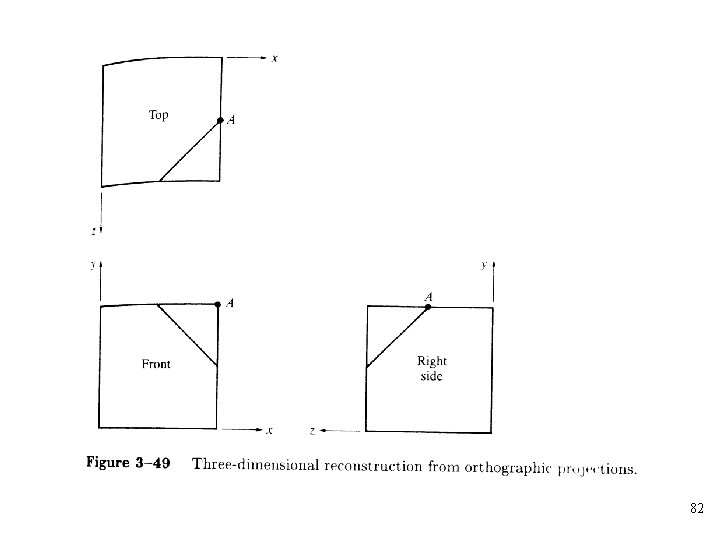82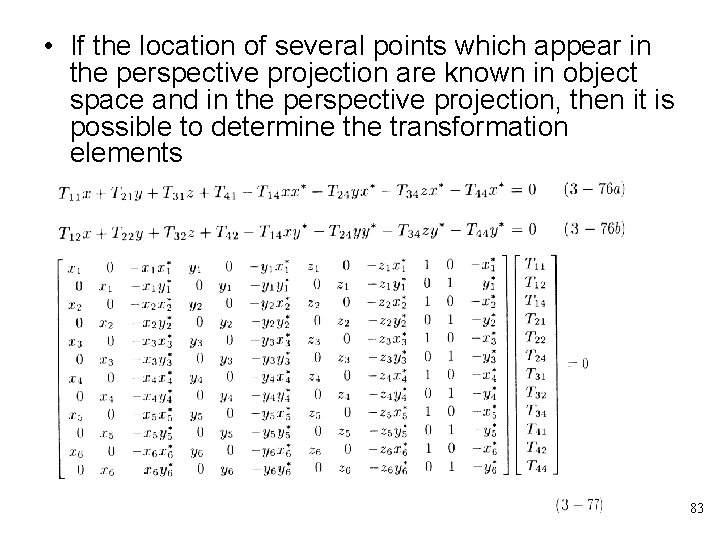• If the location of several points which appear in the perspective projection are known in object space and in the perspective projection, then it is possible to determine the transformation elements 8384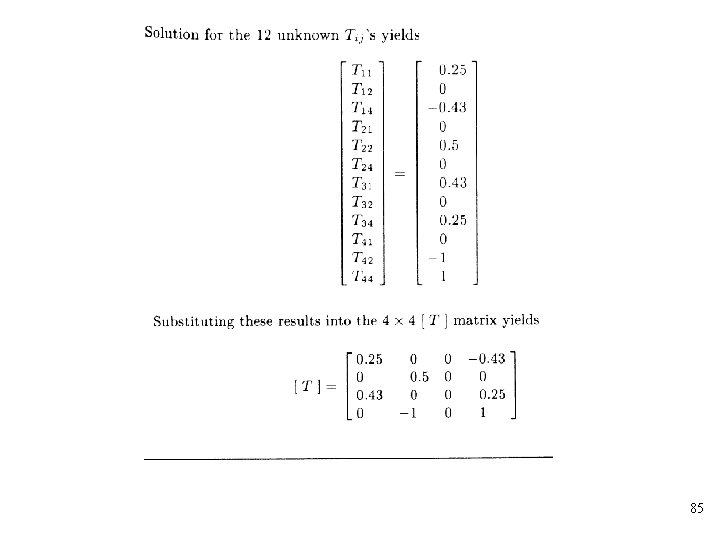85• Project I: Please show the effect of transformation, such as translation, rotation, reflection, parallel projection and perspective projection on a cube with one corner cut off. 86